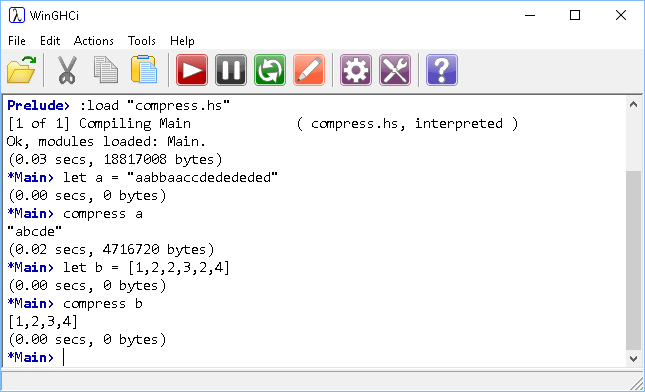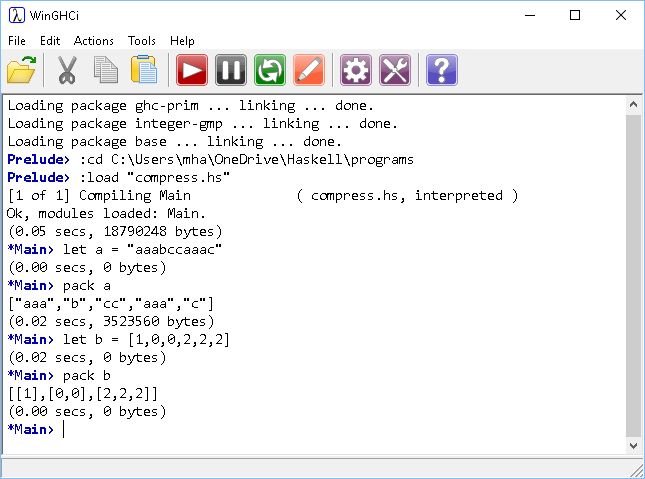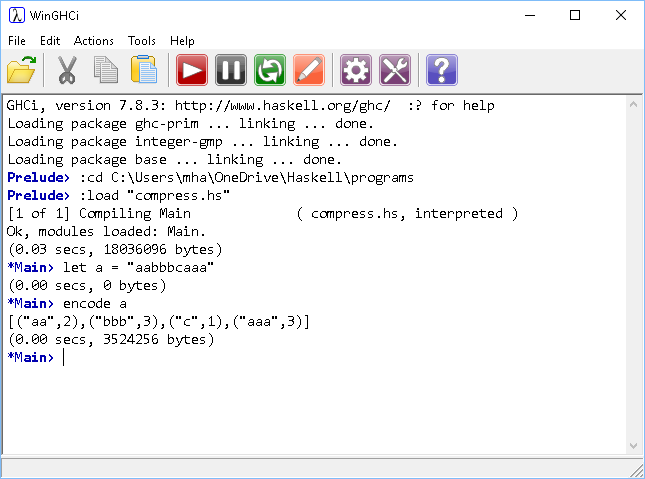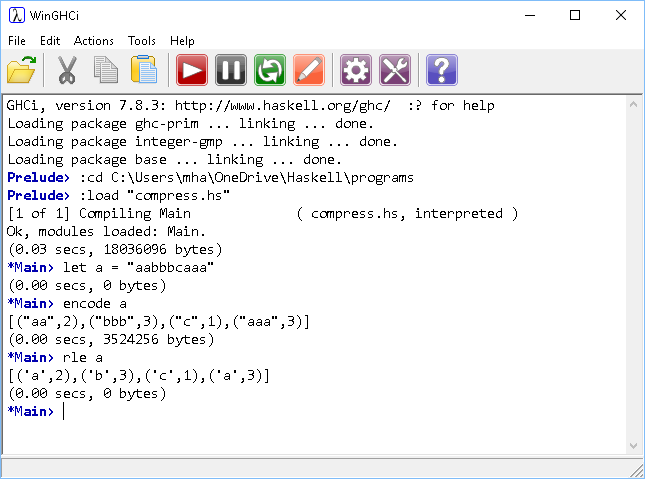# Introduction To Haskell Squishing Lists

## Introduction

This page describes some solutions to several of the problems on the Haskell Wiki, https://wiki.haskell.org/99_questions. The main theme is compressing items in lists and working towards run length encoding of a string.

## Example 1 - Eliminating Duplicate Items

The starting point is to work out a method to remove duplicates from a list. One way to do this is to start a completely new list. Then, take an item at a time from the original list and add it to the new list. If the item we pick is already in the new list, we don't add it.

```elimDupes [] acc = acc                          -- empty
elimDupes (x:xs) acc
| elem x acc = elimDupes xs acc         -- head in acc
| otherwise = elimDupes xs (acc ++ [x]) -- head not in acc
compress l = elimDupes l []
```

The elimDupes function takes two arguments. The first is the list we want to compress, the second is an accumulator for the list we want to return. If the list is empty, the accumulator is returned.

The second definition uses pattern matching. If the head of the list is contained in our accumulator list, we make a recursive call to the function passing the accumulator and the tail of the list (not adding the item to our new list). The otherwise guard is for when the head of the list is not in the accumulator. This item is added to the accumulator before a recursive call is made with the tail of the list.In terms of compression, this function makes an index of the unique items in the input list.

## Example 2 - Make Sublists Of Consecutive Duplicates

For run-length encoding, we would need to know how long the runs of each character are in the list. One step towards that would be to restructure the list so that runs of a single character are turned into a lists.

```pack [] = []
pack (x:xs) = (x:(takeWhile (==x) xs)):pack (dropWhile (==x) xs)
```

Here, the takeWhile and dropWhile functions are really useful. You use takeWhile like you do the filter function. It returns a list consisting of items taken from the front of a list while a condition remains true. The dropWhile function drops items from the front of a list while a condition remains true.

We are using recursion in this solution. If an empty list is supplied as an argument, an empty list is returned. If the list consists of items, a new list is made. Each item in the new list will itself be a list. Work from left to right and it isn't too hard to follow the logic of this. The head of the new list is a list made of the head of the old list and all of the items from the front of the tail that match it,

(x:(takeWhile (==x) xs))

The tail of this new list is taken by repeating the process with whatever is left. That means we have to drop all of those items that we previously took from the list,

pack (dropWhile (==x) xs)## Example 3 - Run-Length Encoding

This problem was to take the sublists produced by the pack function and create a list of tuples containing the item and the run length.

```encode l = zip (pack l) (map (length) (pack l))
```

This gives us the following,Nearly there, but we just want the character, not the full list.

```pack [] = []
pack (x:xs) = (x:(takeWhile (==x) xs)):pack (dropWhile (==x) xs)

encode l = zip (pack l) (map (length) (pack l))

delist ((x:xs),y) = (x,y)

rle l = map delist (encode l)
```

The delist function uses pattern matching for both lists and tuples. It returns a new tuple where the head of the list of the first item in the tuple is used as the new first item.## Challenge

An obvious starting point is to work on a function for reversing this process. Work out how to recreate the string that was encoded in the last example.